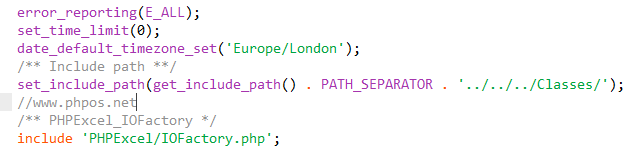• 網站導航
• 投稿方式
• 投訴建議
• 版權聲明
• 關于我們

WWW.2L3.NET

# DEDE批量導入excel數據到后臺文章系統的開發教程

1）開發前的準備：2) 開發插入數據代碼：

```<?php
require_once('/../dedecms/include/common.inc.php');

error_reporting(E_ALL);
set_time_limit(0);
date_default_timezone_set('Europe/London');
/** Include path **/
set_include_path(get_include_path() . PATH_SEPARATOR . '../../../Classes/');
/** PHPExcel_IOFactory */
include 'PHPExcel/IOFactory.php';
if(!empty(\$_GET)){
\$typeid = \$_GET['typeid'];
\$dopost = \$_GET['do'];
if(\$dopost == "exdata"){
if(!empty(\$_GET['n'])){
\$inputFileName = './'.\$_GET['n'].'.xlsx';
\$sheetData = \$objPHPExcel->getActiveSheet()->toArray(null,true,true,true);
\$rowarr=array();
while (\$rowall = \$dsql->GetArray('all')) {
\$rowarr[]=\$rowall;
}
foreach (\$sheetData as \$v) {
foreach (\$rowarr as \$vt) {
if(in_array(\$v['B'],\$vt)&&\$v['A']==\$vt['bio1']){
echo "貨號為：".\$v['B']."<br>廠商為：".\$v['A']."<br>的數據已在表中，請在excel文件里面刪除這一條后再添加！";
exit;
}
}
}
//附加表插入數據前處理
\$row = \$dsql->GetOne("select aid,bio2 from `dede_addonshop` order by aid desc");
if(!empty(\$row)){
\$aid = \$row['aid'];
\$bio2 = \$row['bio2'];
}else{
\$aid = 0;
\$bio2 = '';
}
//處理重復問題
if(\$bio2==\$sheetData[count(\$sheetData)]['B']){
ShowMsg("不能重復添加內容",'javascript:;');
exit;
}
//主表插入數據前處理
\$arcrow = \$dsql->GetOne("select id from `dede_archives` order by id desc");
if(!empty(\$arcrow)){
\$arcid = \$arcrow['id'];
}else{
\$arcid = 0;
}
//微表插入數據前處理
\$tinyrow = \$dsql->GetOne("select id from `dede_arctiny` order by id desc");
if(!empty(\$tinyrow)){
\$tinyid = \$tinyrow['id'];
}else{
\$tinyid = 0;
}
//找出最大的id
\$id = max(\$aid,\$arcid,\$tinyid);
//字段數量
\$fieldnum = count(\$sheetData);
\$fields = \$fieldvalue = '';
for (\$i=0; \$i < \$fieldnum; \$i++) {
\$fields .= \$sheetData[\$alphalpha[\$i]]. ',';
}
\$fields = substr(\$fields, 0,-1);
//遍歷數組
foreach (\$sheetData as \$value) {
\$pubdate = GetMkTime(GetDateTimeMk(time()));
\$click = mt_rand(50, 200);
if(\$value['A']=='bio1'|\$value['A']=='廠商'){
continue;
}
\$id = \$id+1;
//獲取字段值\$value['A'];
for (\$i=0; \$i < \$fieldnum; \$i++) {
\$fieldvalue .= " ,'".\$value[\$alphalpha[\$i]]."' ";
}
//標題
\$C = trim(\$value['C']);
//保存到主表
\$senddate = time();
\$arcquery = "INSERT INTO `dede_archives`(id,typeid,title,mid,channel,pubdate,senddate,click,ismake)VALUES ('\$id','\$typeid','\$C','1','6','\$pubdate','\$senddate','\$click','-1');";
\$dsql->ExecuteNoneQuery(\$arcquery);
//保存到附近加表
VALUES ('\$id','\$typeid'{\$fieldvalue});";
\$dsql->ExecuteNoneQuery(\$query);
\$fieldvalue = '';
//保存到微表
\$tinyquery = "INSERT INTO `dede_arctiny`(id,typeid,channel,mid,senddate)VALUES ('\$id','\$typeid','6','1','\$senddate');";
\$dsql->ExecuteNoneQuery(\$tinyquery);
}
\$num = count(\$sheetData)-2;
ShowMsg("恭喜，成功插入   ".\$num."   條數據！",'javascript:;');
}
}
}else{
echo "密碼或文件名錯誤！您無權做任何操作！";
}
?>```

1）第一步先判斷問號后面是不是為空，即dopost=exdata&typeid=6&n=test1字符串是否為空，如果這個字符串都是空的話，其它，就不用操作了，直接通過出程序，顯示：echo "密碼或文件名錯誤！您無權做任何操作！";

```\$typeid = \$_GET['typeid'];
\$dopost = \$_GET['do'];```

3）插入數據庫表時最大數據id的判斷

3）遍歷數組\$sheetData插入數據GGpoker